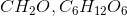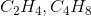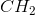# SAT II Chemistry : Empirical and Molecular Formulas

## Example Questions

### Example Question #1 : Empirical And Molecular Formulas

Vanillin is composed ofcarbon,oxygen, andhydrogen.

What is the empirical formula of vanillin?

Refer to the following table for the atomic masses of the elements shown:Explanation:

In order to calculate the empirical formula of vanillin from the given percent composition data, we can pretend we have a 100 g sample vanillin and from there estimate how many grams of each element make up the sample. For example, Vanillin iscarbon; therefore, the estimated sample would contain 66.3 g of carbon. After doing this for each of the elements vanillin contains, we can use the atomic mass of carbon to find how many moles of carbon make up the sample. We then do the same for each element that composes vanillin. See calculations below:After finding the number of moles of each element in the hypothetical 100 g sample, we look at the ratio between the moles of each element by dividing all the samples by smallest number of moles. After finding the ratios of the samples, we are able to use the empirical formula of the vanillin by rounding up to the nearest whole number for each sample. If the ratios are not close enough to round up (like in this example), we multiply the ratios all by the same number until each number is equal to a whole number nicely. See calculations below:We need whole numbers, so multiply each of the resulting numbers by 3 to get rid of the fractions.This gives usas the final answer.

### Example Question #2 : Empirical And Molecular Formulas

Which pair of formulas represents the empirical formula and molecular formula for a certain compound?To find an empirical formula, take a molecular formula and divide the subscript of each element by the greatest common factor of all the subscripts. In this case, the only pair that works is, which can be verified by dividing the coefficients of the molecular formula by 6. Note thatis not correct because neither of the formulas is an empirical formula; they are both possible molecular formulas for a compound with the empirical formula.